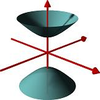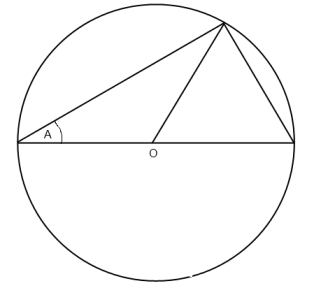#### You may also like### A Close Match

Can you massage the parameters of these curves to make them match as closely as possible?### Prime Counter

A short challenge concerning prime numbers.### The Right Volume

Can you rotate a curve to make a volume of 1?

# Trig Identity

##### Age 16 to 18Challenge LevelIn this diagram $O$ is the centre of a unit circle (i.e. a circle with radius 1). Use it to find two trigonometrical relationships between $A$ and $2A$.

Did you know ... ?

Trigonometrical expressions can often be derived algebraically or geometrically. In more advanced applications the functions of trigonometry are defined as infinite power series rather than geometric ratios.